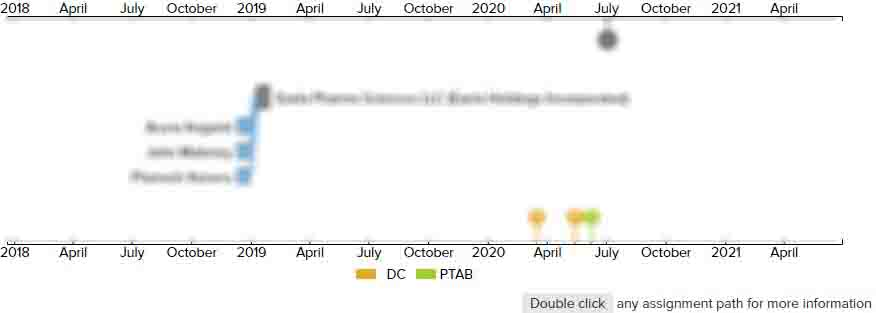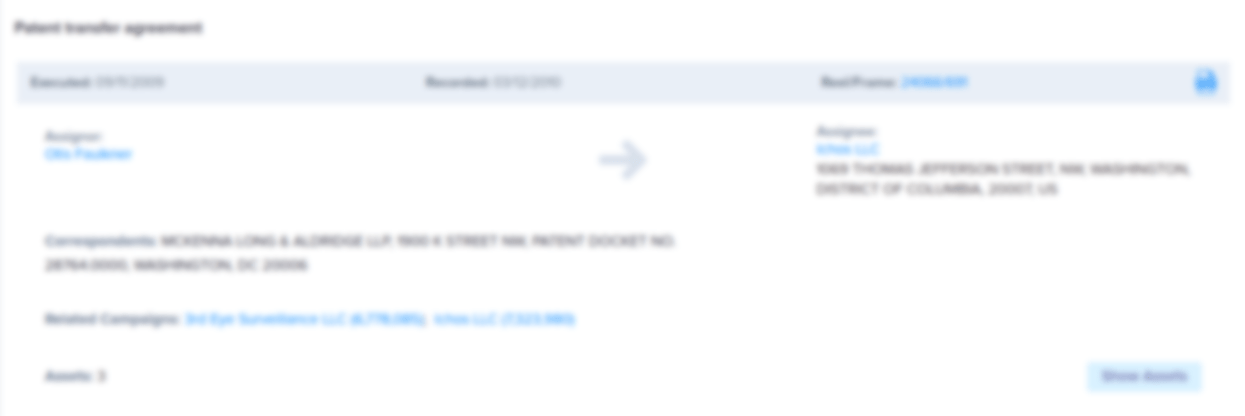# Quantization for hybrid video coding

CAFC
• US RE46,777 E1
• Filed: 02/02/2016
• Issued: 04/03/2018
• Est. Priority Date: 03/13/2007
• Status: Active Grant
##### First Claim
Patent Images

1. A method for coding a video signal using hybrid coding, comprising:

• reducing temporal redundancy by block based motion compensated prediction in order to establish a prediction error signal;

performing quantization on samples of the prediction error signal or on coefficients resulting from a transformation of the prediction error signal into the a frequency domain to obtain quantized values, representing quantized samples or quantized coefficients respectively, wherein the prediction error signal includes a plurality of subblocks each including a plurality of quantized values;

calculating a first quantization efficiency for the quantized values of at least one subblock of the plurality of subblocks;

setting the quantized values of the at least one subblock to all zeroes;

calculating a second quantization efficiency for the at least one subblock while all of the quantized values are zeroes;

selecting which of the first and second quantization efficiencies is a higher efficiency; and

selecting, for further proceeding, the at least one subblock with the quantized values prior to setting the quantized values of the at least one subblock to all zeroes if the first quantization efficiency is higher and selecting the at least one subblock with the quantized values set to zero, for further proceeding, if the second quantization efficiency is higher.

• ##### 0 Assignments
Timeline View
Assignment View×
×